EXPERIMENT NO.11

Broad side linear array with uniform spacing and amplitude

 Time ( min) Content Learning Aid / Methodology Faculty Approach Typical Student Activity Skill / Competency Developed 10 Relevance and significance of experiment Chalk & Talk , Presentation Introduces, Facilitates, Monitors Listens, Participates, Discusses Knowledge, Communication, intrapersonal 20 Explanation of experiment Chalk & Talk , Presentation Introduces, Facilitates, Explains Listens Knowledge, Communication, intrapersonal, Application 20 Calculations N/A Explains, Monitors Participates, Discusses Knowledge, Communication, Intrapersonal, Application 60 Simulation Demonstration Explains, Monitors Participates, Discusses Debate, comprehension, Hands on experiment 10 Results and conclusions Keywords Lists, Facilitates Listens, Participates, Discusses Knowledge, Communication, intrapersonal, Comprehension

Practical Session Plan

Title: Broad side linear array with uniform spacing and amplitude

Objectives: Model the Broad side Array to analyze the performance parameters

Aim:

Â· To sketch the radiation pattern of Broad side Array with uniform spacing & amplitude

Â· To compute performance parameters

Apparatus: PC, EM simution software

Theory:

Antenna Array:

Usually the radiation pattern of a single element is relatively wide, and each element provides low values of directivity (gain). In many applications it is necessary to design antennas with very directive characteristics (very high gains) to meet the demands of long distance communication. This can only be accomplished by increasing the electrical size of the antenna. Enlarging the dimensions of single elements often leads to more directive characteristics. Another way to enlarge the dimensions of the antenna, without necessarily increasing the size of the individual elements, is to form an assembly of radiating elements in an electrical and geometrical configuration. This new antenna, formed by, is referred multielements to as an array. In most cases, the elements of an array are identical. This is not necessary, but it is often convenient, simpler, and more practical. The individual elements of an array may be of any form (wires, apertures, etc.).

The total field of the array is determined by the vector addition of the fields radiated by the individual elements. This assumes that the current in each element is the same as that of the isolated element (neglecting coupling). This is usually not the case and depends on the separation between the elements. To provide very directive patterns, it is necessary that the fields from the elements of the array interfere constructively (add) in the desired directions and interfere destructively (cancel each other) in the remaining space. Ideally this can be accomplished, but practically it is only approached.

In an array of identical elements, there are five controls can be used to shape the overall pattern of the antenna. These are:

1. The geometrical configuration of the array. (Linear, circular, rectangular etc.)

2. The relative displacement between each element.

3. The excitation amplitude of the individual elements.

4. The excitation phase of the individual elements.

5. The relative pattern of individual elements.

Linear array: If the antenna elements are equally spaced along a straight line known as linear array.

Uniform Linear array: AUniform Linear array is one in which the elements are fed with a current of equal magnitude with uniform progressive phase shift.

Broadside array is one in which number of identical parallel antennas are set up along a line drawn perpendicular to their respective axes as shown in fig 1(a).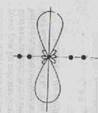In broadside array, individual antennas are equally spaced along a line and each element is fed with equal magnitude, all in same phase. This arrangement fires in broadside directions i.e. perpendicular to the line of array axis, there are maximum radiations and relatively a little radiations in other directions and hence the radiation pattern of broadside array is bidirectional as shown in fig 1(b)., which radiates equally well in either direction of maximum radiations.

In broadside array, it is desired to have maximum radiation of an array directed normal to the axis of the array (broadside=90Â°) to optimize the design, the maxima of single element can be accomplished by the choice of the radiators and those of array factor by the proper separation and excitation of the individual radiators.

For n-element linear array with uniform amplitude and spacing normalised Array factor is given as: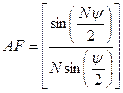â€¦â€¦â€¦â€¦.. (1)

Maximum Array factor occurs when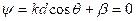Since, it is desired to have the maximum directed toward Î¸ = 90Âº, thenTo have maximum of AF directed broadside to the axis of array, it is necessary that all the elements have same phase excitation. The separation between the elements can be of any value. To ensure that there are no maximum in other directions, the separation between the elements should not be equal to multiples of a wavelength (d â‰  nÎ», n=1,2,3â€¦â€¦) when Î²=0. If d =nÎ», n=1,2,3â€¦â€¦ and Î² = 0, then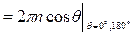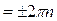This value of Ïˆ, when substituted in equation 1 makes the array factor attain its maximum value.

Procedure:

1. Calculate wavelength of dipole using the formula Î» = c/f , where c= 3*108& fis the frequency.
2. Initially open the notepad and write down the command given below

CM (Comment card)

CE (Comment enable)

EN (End)

Save the file with the extension .nec command

2. Click on the 4nec2 software shortcut icon present on desktop and then click on file option chooses open our saved notepad file.
3. Press control +F4 then window(filename.nec.txt) is opened, OR
4. Click on setting option and choose NEC editor (new) option
5. Click on edit NEC input file (Red colour book icon )
6. Choose symbol option and after choosing custom factor option if we want to do the calculation in meters then take 0.001 value (because 1m=1000mm)
7. Then select save and then click on structure is resized Ok.
8. Then choose geometry option. In type option we are making wire antennas so choose type is wire. Next option is tag which is a voltage source so it should keep as 1. Next is number of segments here we can choose any odd no. E.g. 3, 5, 7, 9, 11, 13........ In X, Y, Z we can write the length of our dipole as here our dipole is on Y axis so we given lambda/2 value on Y axis. Next is the radius of dipole i.e. thickness of conductor.
9. Then click on source/load option and specify voltage.
10. Then click on Frequency/Ground, and then specify the frequency and ground. Select frequency as given frequency, Environment= free space.
11. The next step is calculation of radiation pattern for that click on green book icon on main window for generating output select far field pattern option.
12. If we want to see 3D radiation pattern of antenna then click on 3D option on main window.
13. Take the print out for Geometry, Radiation pattern in 2D and 3D.

Design:

Use 4nec2 software to implement a Broadside array and its polar pattern for n = 6, d = 0.7Î», Î± = 0Â° and f = 300Mhz

f = 300Mhz

Î» = c/f

Î» = 3*108 / 300*106 = 1m.

d= spacing between the adjacent element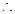d = 0.7 Î» = 0.7 *1m = 0.7m

Simulation steps: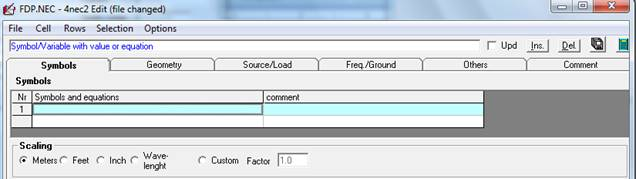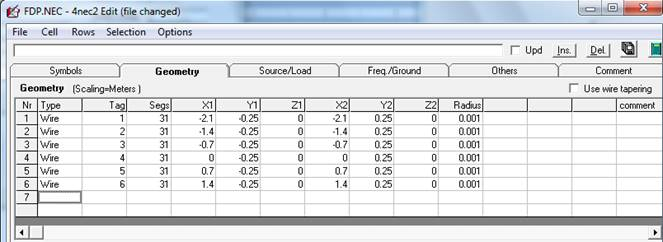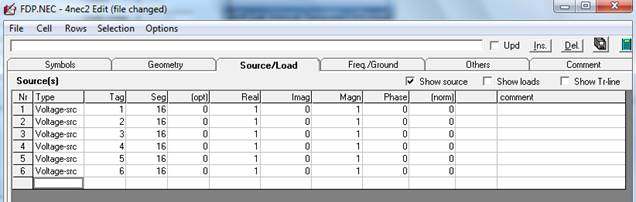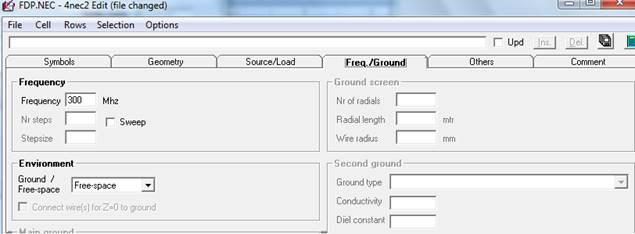Results: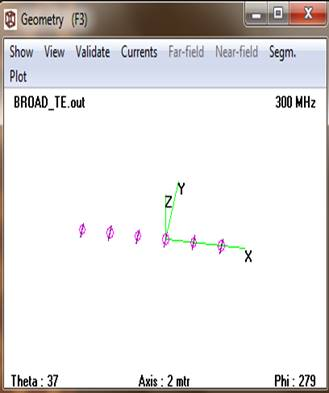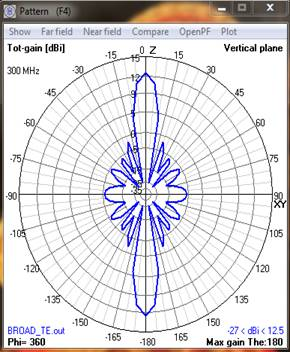Conclusion:

In Broadside array the maximum radiation is perpendicular to axis of antenna array i.e. at 90o and 270o and the maximum radiation can be obtained by increasing number of elements.

Upon completion of experiment students will be able to:

1. Model the Broad side Array.

2. Analyze the performance parameters

Oral Question Bank

Theory Question Bank

 Q. No Description 1 What is an â€œarray factorâ€? 2 What are the types of antenna arrays? 3 Define the length of an array 4 What are the applications of arrays? 5 How to convert broad side array radiation pattern into unidirectional? 6 Define antenna array. 7 What is an array and mention the various forms of antenna arrays? 8 What is array factor or space factor? 9 Define HPBW? 10 Define BWFN? 11 An array consisting a no. of equidistant dipoles of equal size, fed with the same currents and phase is called:(a) end fire array (b) back fire array (c) broad side array (d) binomial array 12 Define FSLBW? 13 What is a collinear array? 14 What are the characteristics of broad side array? 15 Which are the controlling parameters of the overall pattern of antenna array? 16 Why antenna arrays are required? 17 What is uniform linear array? 18 What is the effect of changing the number of element on the radiation pattern of an antenna array? 19 What is the effect of changing the spacing between adjacent elements on radiation pattern of an antenna array? 20 Write the principle of pattern multiplication?